# How to calculate end date from start date and duration in Excel?

Spreadsheets allow Excel's powerful data visualisation and analysis software to manage, store, and display massive amounts of data in a graphical format. Excel is a powerful data visualisation and analysis software. Each individual piece of data is saved inside of a cell, which is a square or rectangular block.

Creating graphs using this tool allows users to visualise patterns, compare data, and gain insights from the data by utilising functions and formulas. Its primary application is in the field of accounting; however, anyone can use it for a wide variety of jobs so long as the requirements are met. Let's have a look at the formula for determining the end date based on the start date and the duration.

In the event that you have a list of start dates and durations, the next step is to compute the end date based on the start date and the duration. How might one go about finding a solution to this task using Excel? Please follow the rules that are given below in order to calculate the end date based on the start date and the (duration) number of weeks.

Let’s understand step by step with an example.

## Step 1

In the first step, let’s assume we have a sample data for calculating end date from start date and duration in weeks as shown in the below screenshot for the same.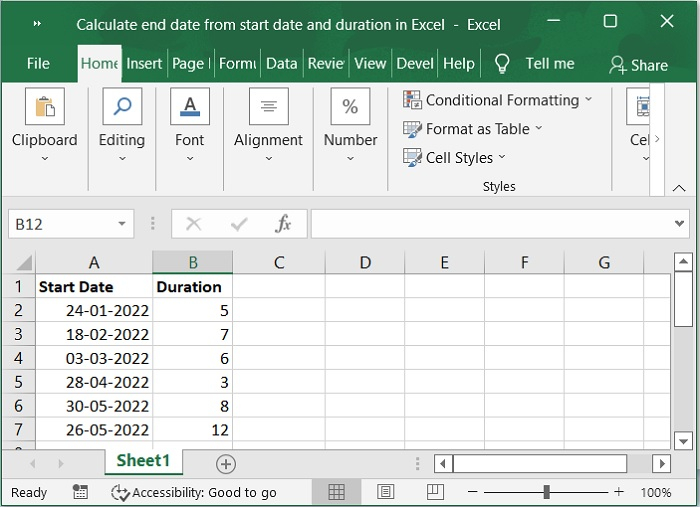## Step 2

In the next step, please enter the following formula into a cell that is blank if you want to determine the end date based on the start date and the number of weeks. Please refer to the below screenshot for the same.

=IF(A2,A2+(B2*7),””)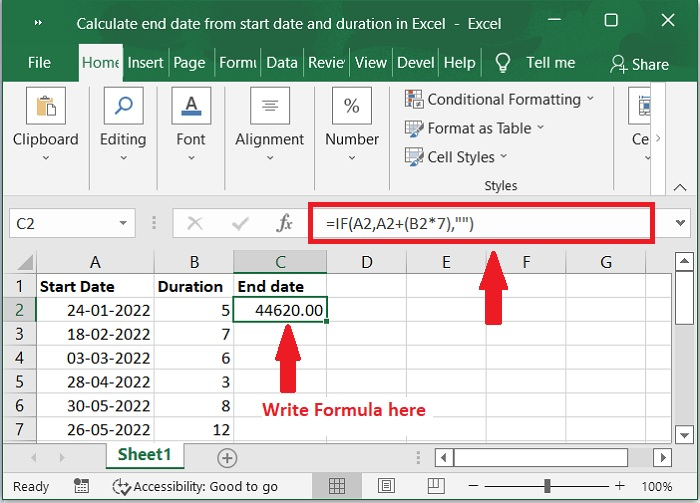Important Note − In the above formula, A2 is start date and B2 is duration of weeks.

## Step 3

Then, move the fill handle to the cells where you wish to apply this formula, and a list of numbers will appear, as shown in the screenshot that follows.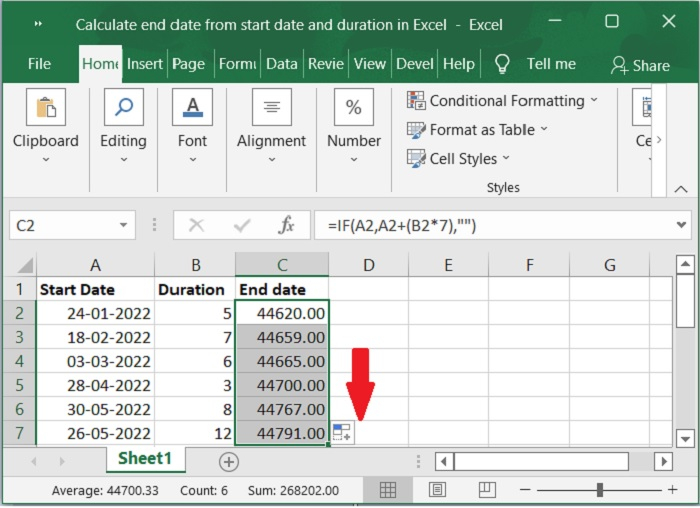## Step 4

The next step is to convert the numerical values into date format by selecting the Home tab, then select Short Date from the General drop-down menu. Please check out the below screenshot for the same.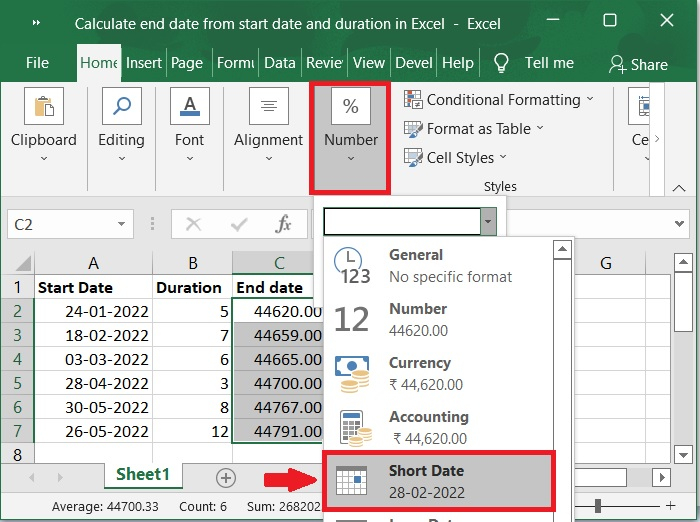## Step 5

In addition, the numerical values have been displayed in date format. Please refer to the below screenshot for the same.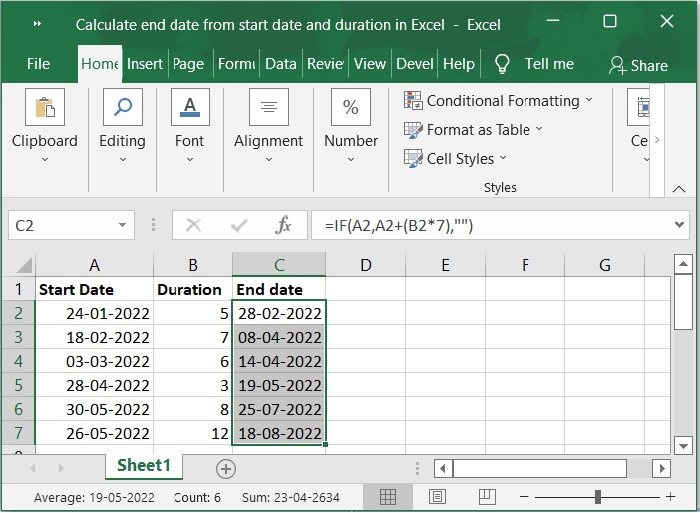Important Note − In this article, we have explained duration in week only. If you want to calculate duration of month and year, please use the below formulas.

## Formula of Month

=DATE(YEAR(A2),MONTH(A2)+B2,DAY(A2))


## Formula of Year

=DATE(YEAR(A2)+B2,MONTH(A2),DAY(A2))


## Conclusion

We hope this tutorial helped you understand how to calculate end date from start date and duration by using simple formulas in Excel.

Updated on: 03-Feb-2023

926 Views